Constructing a triangle when 2 sides and angle between is known (SAS)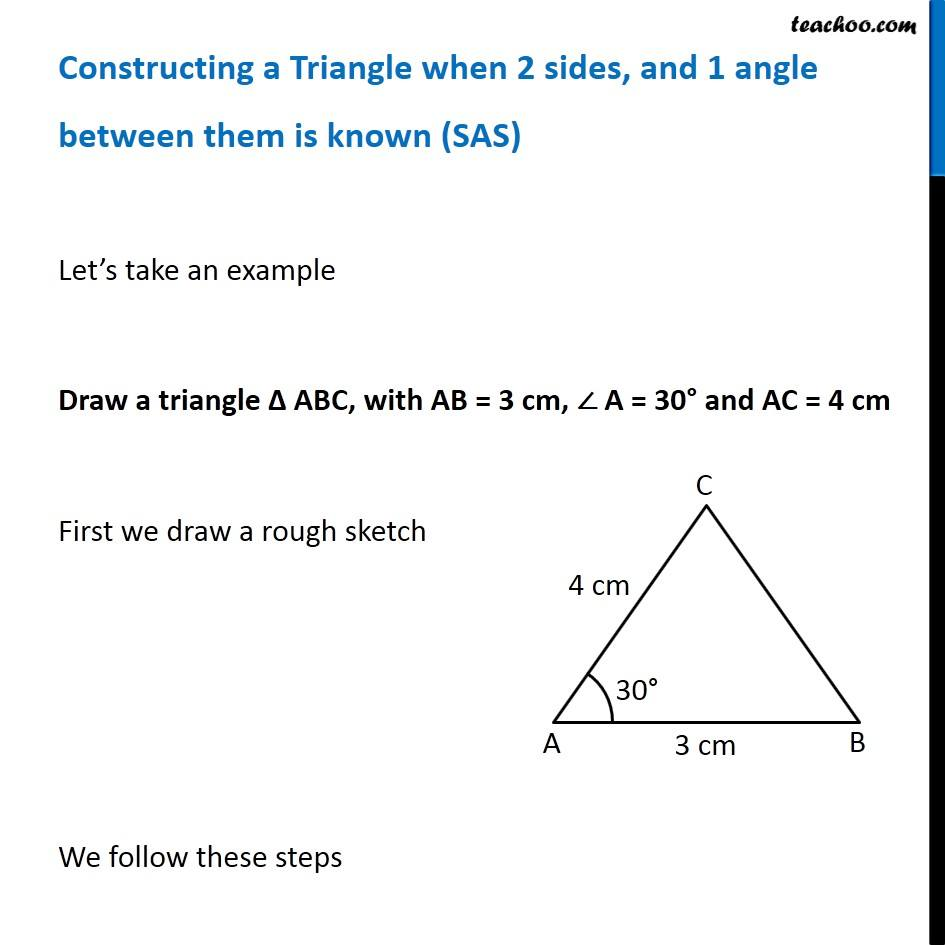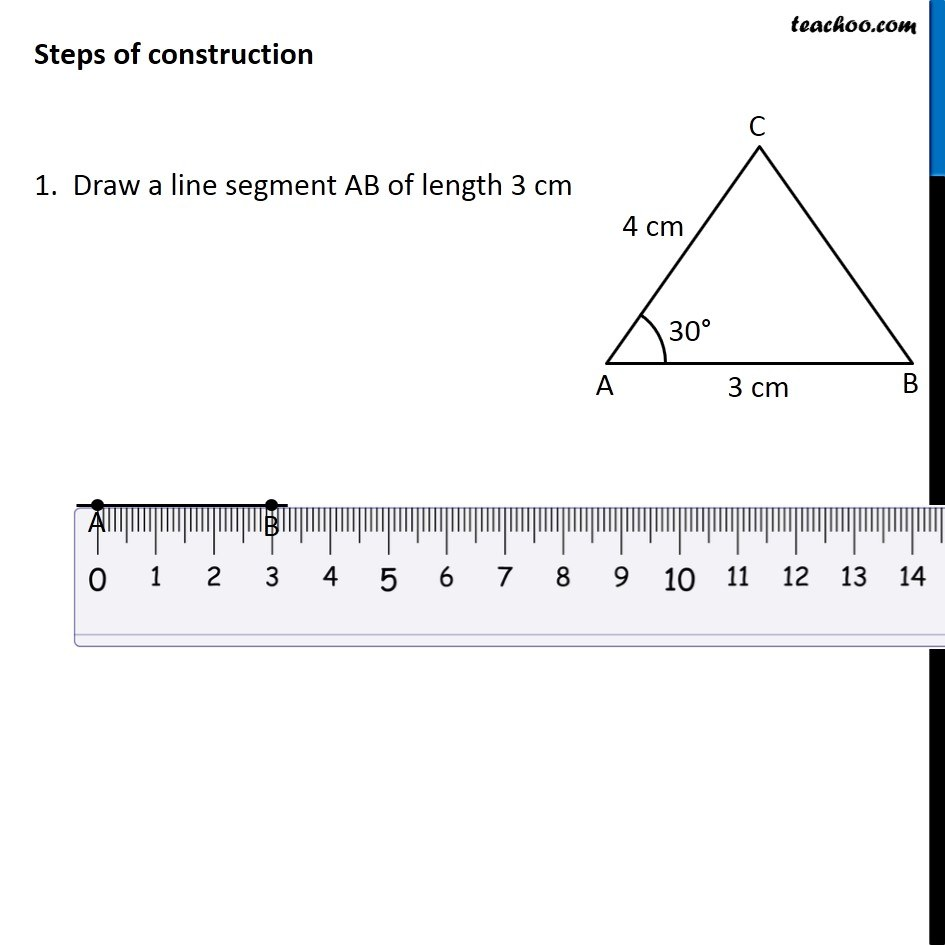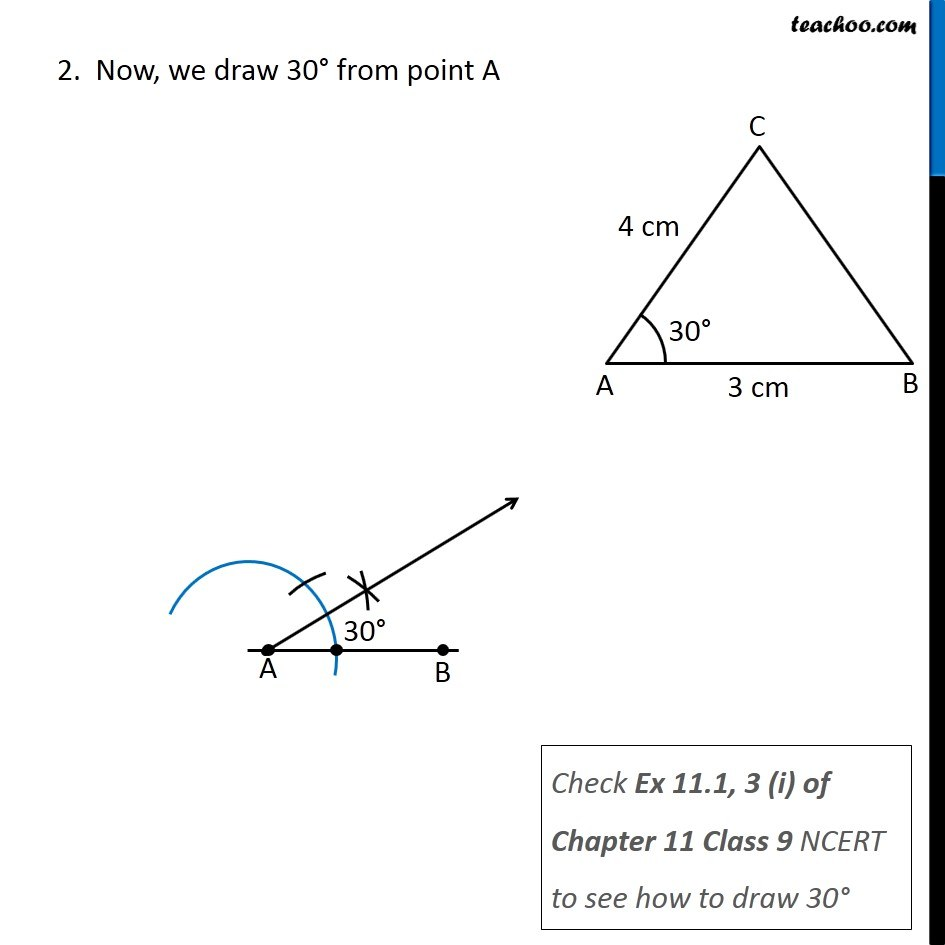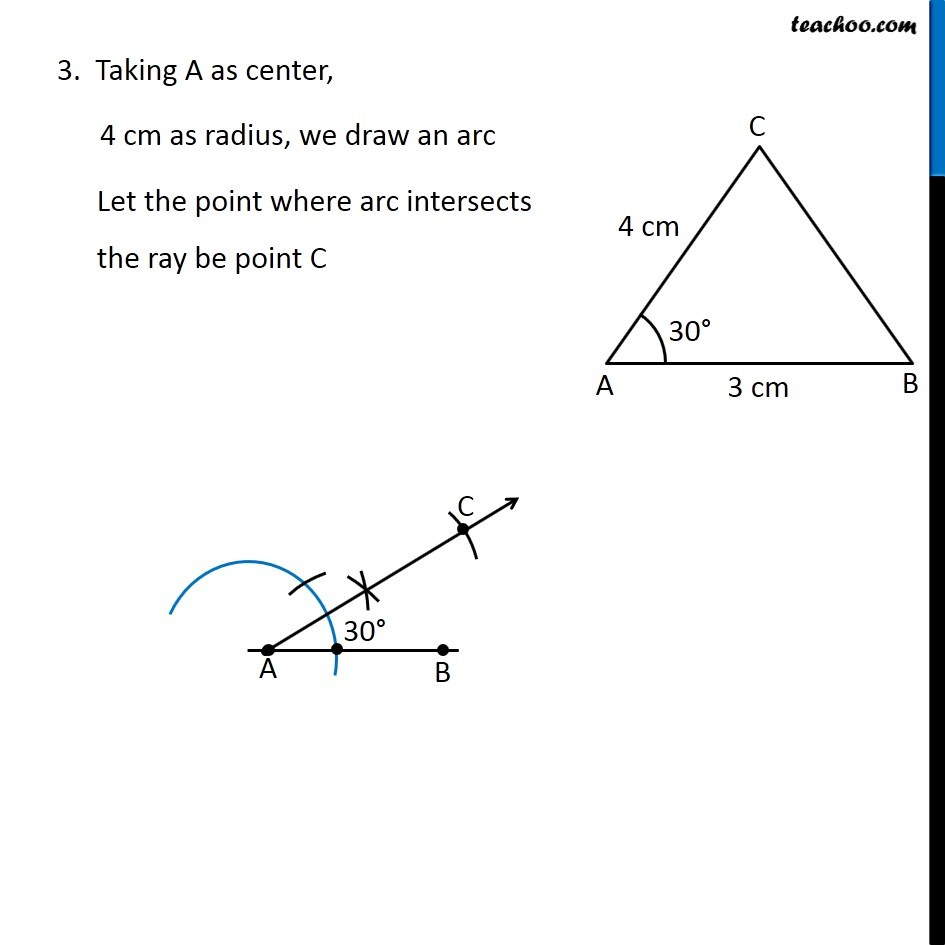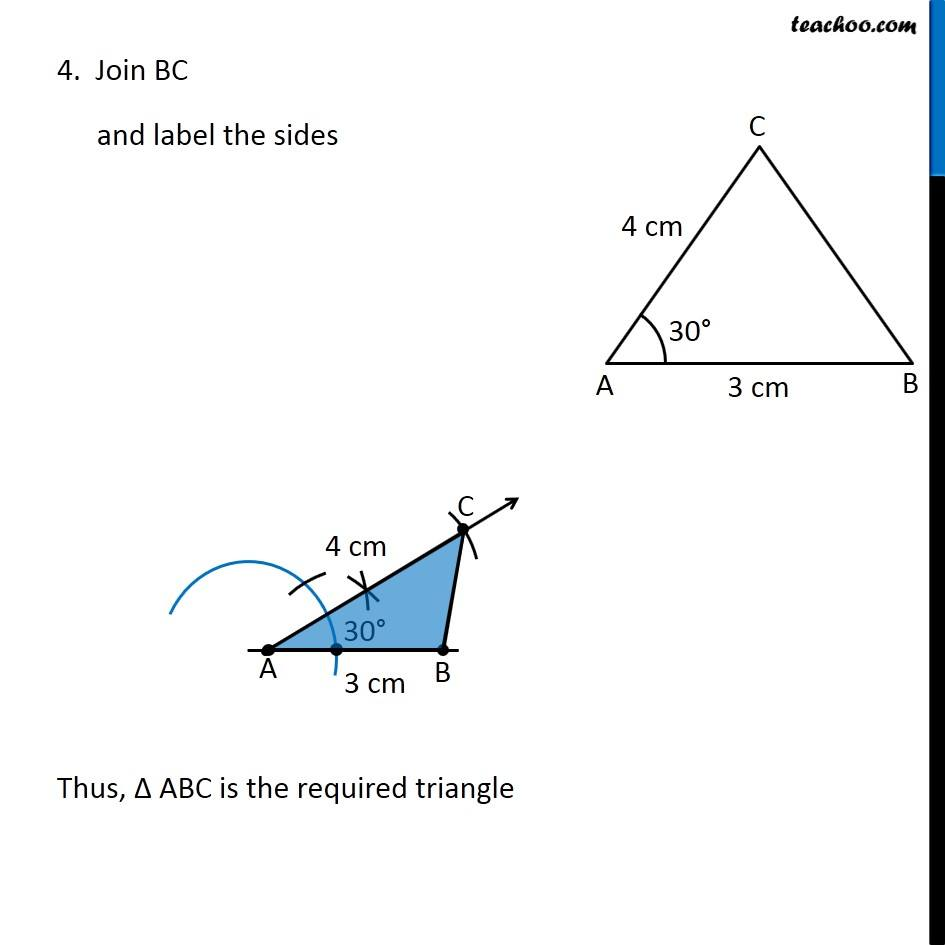Learn in your speed, with individual attention - Teachoo Maths 1-on-1 Class

### Transcript

Constructing a Triangle when 2 sides, and 1 angle between them is known (SAS) Let’s take an example Draw a triangle Δ ABC, with AB = 3 cm, ∠ A = 30° and AC = 4 cm First we draw a rough sketch We follow these steps Steps of construction 1. Draw a line segment AB of length 3 cm 2. Now, we draw 30° from point A Check Ex 11.1, 3 (i) of Chapter 11 Class 9 NCERT to see how to draw 30° 3. Taking A as center, 4 cm as radius, we draw an arc Let the point where arc intersects the ray be point C 4. Join BC and label the sides Thus, Δ ABC is the required triangle